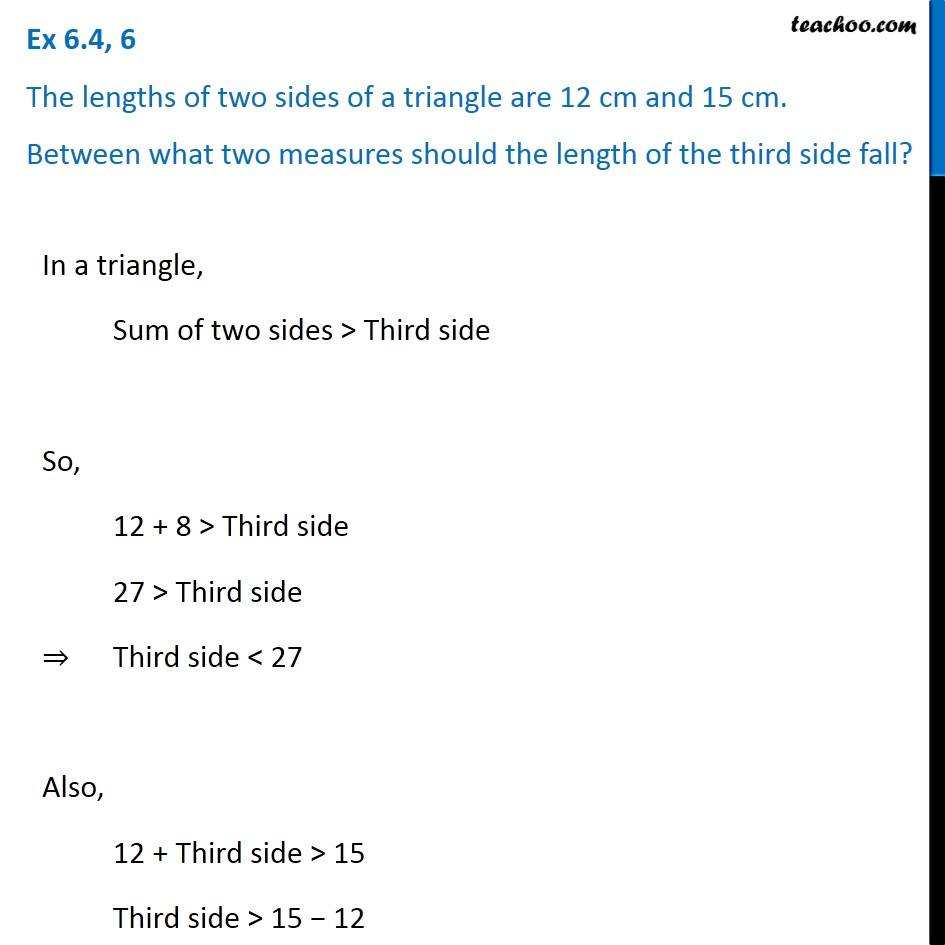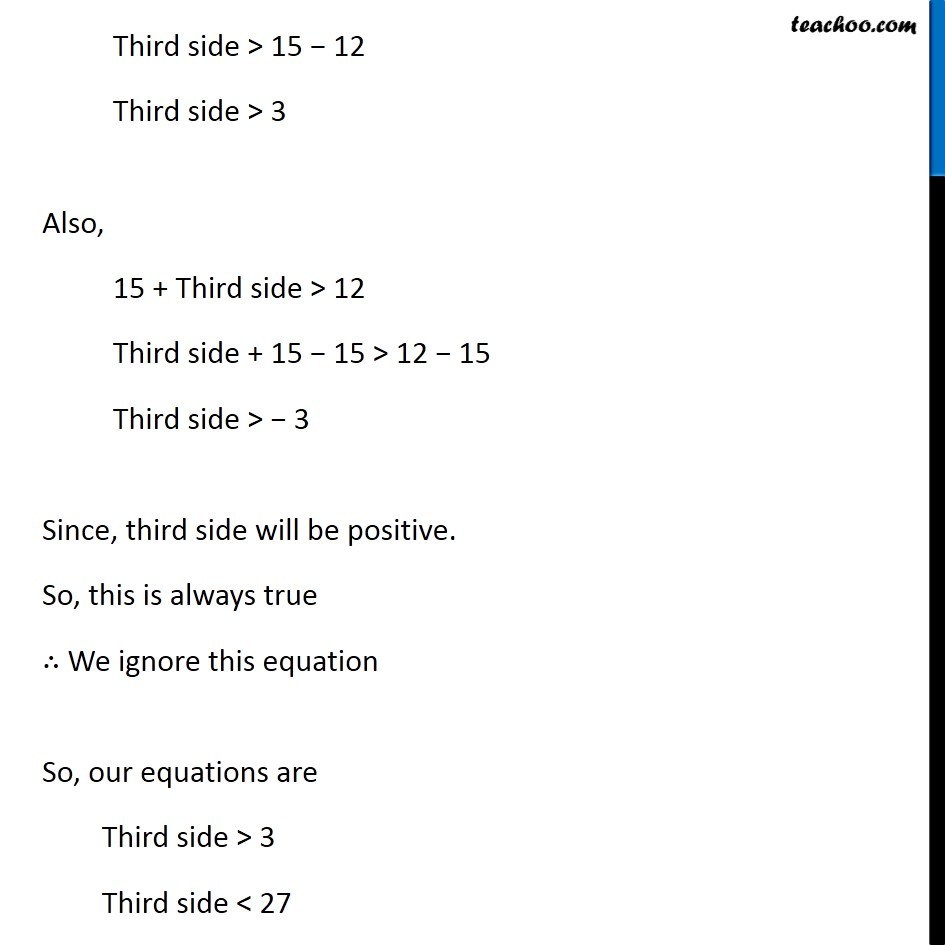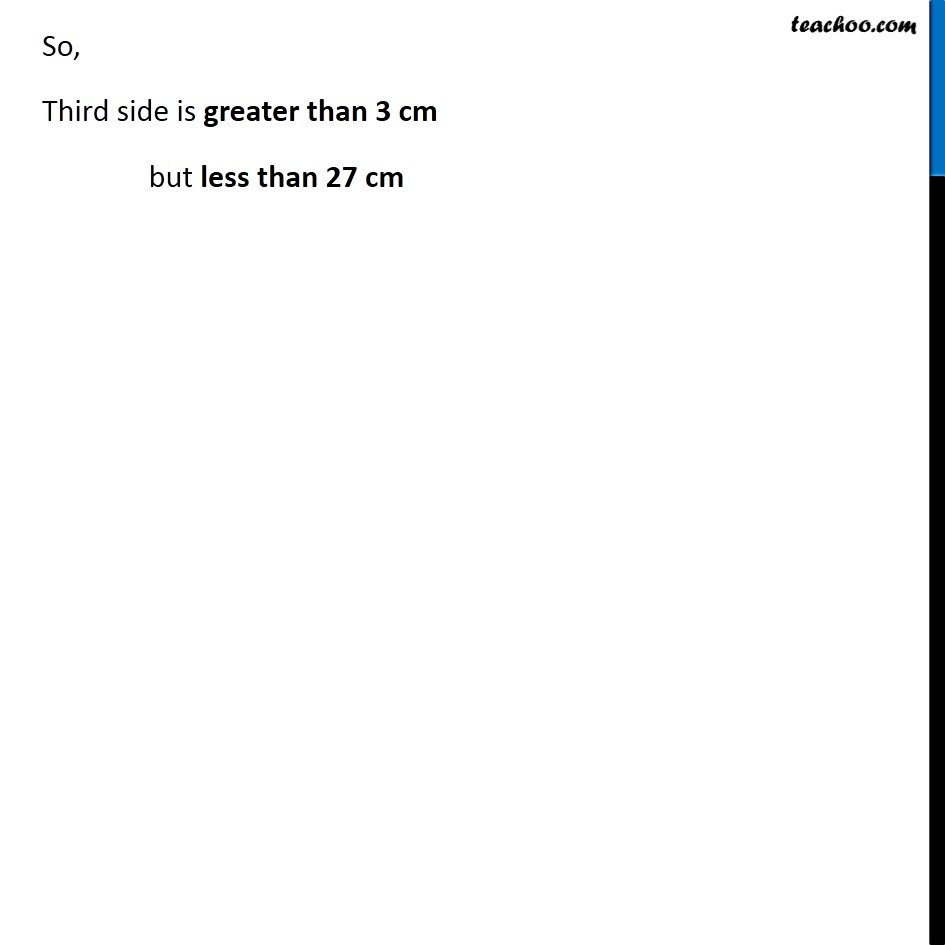Sum of lengths of two sides of a triangle

Chapter 6 Class 7 Triangle and its Properties
Concept wise### Transcript

Ex 6.4, 6 The lengths of two sides of a triangle are 12 cm and 15 cm. Between what two measures should the length of the third side fall? In a triangle, Sum of two sides > Third side So, 12 + 8 > Third side 27 > Third side ⇒ Third side < 27 Also, 12 + Third side > 15 Third side > 15 − 12 Third side > 15 − 12 Third side > 3 Also, 15 + Third side > 12 Third side + 15 − 15 > 12 − 15 Third side > − 3 Since, third side will be positive. So, this is always true ∴ We ignore this equation So, our equations are Third side > 3 Third side < 27 So, Third side is greater than 3 cm but less than 27 cm